StatLect

# Covariance matrix of the maximum likelihood estimator

The asymptotic covariance matrix of a maximum likelihood estimator (MLE) is an unknown quantity that we need to approximate when we want to build confidence intervals around the point estimates obtained with the maximum likelihood method.

It is not to be confused with the MLE of the covariance matrix of a distribution (check this page).

This lecture presents three popular estimators that can be used to approximate the asymptotic covariance matrix of the MLE:

1. the outer product of gradients (OPG) estimator;

2. the Hessian estimator;

3. the Sandwich estimator.## The setting

Letbe the realizations of the firstterms of an IID sequence.

Suppose that a generic term of the sequence has probability density (or mass) functionwhereis an unknown vector of parameters.

The maximum likelihood estimator ofis## Asymptotic covariance matrix

As proved in the lecture on maximum likelihood, under certain technical assumptions the distribution ofis asymptotically normal.

In particular, the distribution ofcan be approximated by a multivariate normal distribution with meanand covariance matrixwhere:

•is the log-likelihood of a single observation from the sample, evaluated at the true parameter;

• the gradientis the vector of first derivatives of the log-likelihood;

•is the so-called asymptotic covariance matrix.

Note that we divide bybecause the asymptotic covariance matrix is the covariance matrix of, while we are interested in the covariance of.

## Information equality

Under the technical assumptions mentioned previously, the information equality holds:where the Hessian matrixis the matrix of second-order partial derivatives of the log-likelihood function.

## Outer product of gradients (OPG) estimator

The first estimator of the asymptotic covariance matrixis called outer product of gradients (OPG) estimator and it is computed as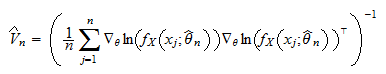It takes its name from the fact that the gradientis a column vector, its transpose is a row vector, and the product between a column and a row is called outer product.

Provided some regularity conditions are satisfied, the OPG estimatoris a consistent estimator of, that is, it converges in probability to.

Proof

We provide only a sketch of the proof and we refer the reader to Newey and McFadden (1994) for a more rigorous exposition. Provided some regularity conditions are satisfied (see the source just cited), we have the following equality between probability limits: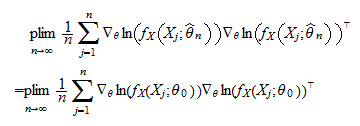wherehas been replaced bybecause, being a consistent estimator, it converges in probability to. Because the sample is IID, by the Law of Large Numbers we have thatNow, the formula for the covariance matrix (see the lecture entitled Covariance matrix) yields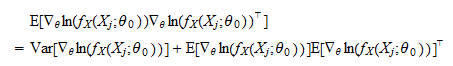But the expected value of the gradient evaluated atis, so that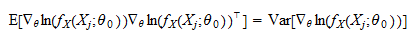Thus,Because matrix inversion is continuous, by the Continuous Mapping theorem we have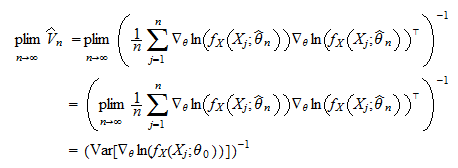which is exactly the result we needed to prove.

## Hessian estimator

The second estimator of the asymptotic covariance matrixis called Hessian estimator and it is computed asUnder some regularity conditions, the Hessian estimatoris also a consistent estimator of.

Proof

Again, we do not provide an entirely rigorous proof (for which you can see Newey and McFadden - 1994) and we only a sketch the main steps. First of all, under some regularity conditions, we have that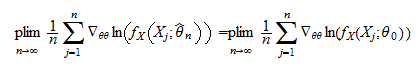wherehas been replaced bybecause, being a consistent estimator, it converges in probability to. Now, since the sample is IID, by the Law of Large Numbers we have that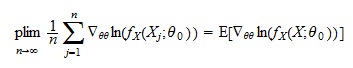By the information equality, we haveTherefore,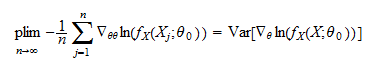Because matrix inversion is continuous, by the Continuous Mapping theorem we havewhich is what we needed to prove.

## Sandwich estimator

The third estimator of the asymptotic covariance matrixis called Sandwich estimator and it is computed aswhereis the OPG estimator andis the Hessian estimator.

Also the Sandwich estimatoris a consistent estimator of.

Proof

This is again a consequence of the Continuous Mapping theorem:where the last equality follows from the consistency of the OPG and Hessian estimators.

## References

Newey, W. K. and D. McFadden (1994) "Chapter 35: Large sample estimation and hypothesis testing", in Handbook of Econometrics, Elsevier.